## 参数设置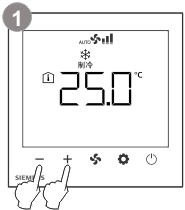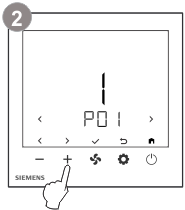或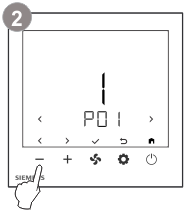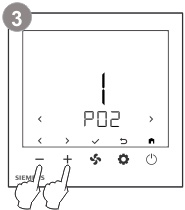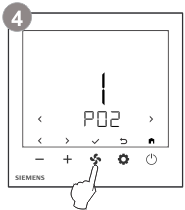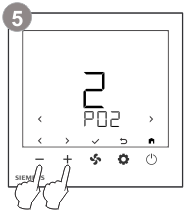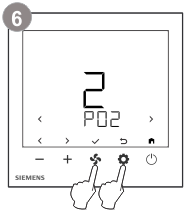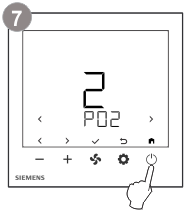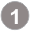同时按下 + 和 - 键 3 秒以上。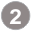初级参数：松开按键且在 2 秒内按下 + 键，直到屏幕显示 Pxx。高级参数：松开按键且在 2 秒内按下 - 键，直到屏幕显示 Pxx。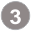按下 + 或 - 键（使用 < 或 > 键）选择参数，所选参数的当前值会显示。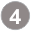按下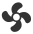键（使用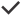键）以编辑所需参数的值。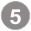在编辑过程中，按下 + 或 - 键增加/减少或选择当前参数的值。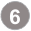按下键（使用键）以确认更改的值，或按下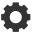键（使用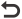键）取消更改。重复步骤 3 到 5，以更改更多参数。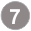按下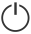键（使用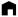键）退出参数设置模式。

## 恢复出厂设置

• 选择参数 P89，并把参数值设置为 1。
• 控制参数的出厂设置将会重新加载。
• 重载过程中，屏幕显示“- - -”。

## 控制参数

P01

0

0 = 两管制

1 = 四管制

P02

1

0 = 仅供热

1 = 仅制冷

2 = 供热制冷自动转换

3 = 供热制冷手动转换

4

3 = 供热制冷手动转换

4 = 供热和制冷

P03

1

0 = 1 速风机

1 = 3 速风机

P04 *

0

0 = 开/关两线阀

1 = 开/关三线阀

P10

P02 ≠ 3

0

0 = 舒适 – 保护

1 = 舒适 – 节能 – 保护

P02 = 3

0

0 = 舒适 – 保护

P11

0

0 = 内置或连接至 X1 端的传感器室内温度

1= 温度设定值

P12

0

0 = ℃（摄氏度）

1 = °F（华氏度）

P13

0

-5…5 K

P14

0

0 = 关闭

1 = 不同单位的温度标准显示 (C/F)

2 = 温度设定值

P15

0

0 = 解锁

1 = 锁定（全锁）

2 = 设定值可调节

P20

5 °C

5 ℃…P21-1 (P21 = 40 ℃ 最大值)

P21

35 °C

(P20 = 5 ℃ 最小值) P20+1…40 ℃

P22

15 °C

0 = 禁用（关闭）

5…40 ℃ (P23)

P23

30 °C

0 = 禁用（关闭）

5 (P22)…40 ℃

P24

0

0 = 禁用

1 = 低速（供热和制冷）

2 = 低速（仅制冷）

P30

0

0 = 前一运行模式

1 = 舒适模式

2 = 保护模式

P31

1

0 = 禁用

1 = 启用

* 此参数值 1 仅在 P01 = 0 时可见。

P40

2 K

0.5…6 K

P41

1 K

0.5…6 K

P42

2 K

0.5…5 K

P50

8 °C

0 = 禁用（关闭）

5...40 °C (P51)

P51

0

0 = 禁用（关闭）

5 (P50)...40 °C

P60

0

0 = 无功能

1 = 外接温度或回风温度传感器

2 = 冷热转换传感器或开关

3 = 开窗探测

6 = 告警触点

7 = 监测外接开关状态

8 = 监测外接传感器状态

9 = 存在探测

10 = 酒店门禁卡

P61 ***

X1 标准位置

0

0 = 开启

1 = 关闭

2 = NTC 3k

3 = NTC 10k

P62 **

16 °C

10…25 ℃

P63 **

28 °C

27…40 ℃

P70

0

0 = 关闭

1…359 分钟

360 = 风机持续运转

P71

0

0 = 禁用

1 = 启用

2 = 重置计数器（P71 自动更改为1）

P89

0

0 = 禁用

1 = 开始重载

** 这些参数仅在P60 = 2 和 P61 = 2 or 3 时可见。

*** 此参数值 0 & 1 仅在 P60 = 2, 3, 6, 7, 9, 10 时可见，同时参数值 2 & 3 仅在 P60 = 1, 2, 8 时可见。

d00

"---" = 无错误

AL1 – 外部错误输入 X1

Er3 - 外接温度或回风温度传感器错误

d01

0 = 不计数

1...990 小时 (分辨率以 10 小时计)

d02

X1 状态

"---" = 未选择功能

0 = 未激活 (DI)

1 = 激活 (DI)

0…49 = 当前温度值 (AI)

-100 = 故障 (AI)

00 = 供热制冷输入短路

100 = 供热制冷输入开路

d03

OPE = Q1 输出强制开启

CLO = Q1 输出强制关闭

d04

OPE = Q2 输出强制开启

CLO = Q2 输出强制关闭

d05

OPE = Q3 输出强制开启

CLO = Q3 输出强制关闭

d06

OPE = Y11 输出强制开启

CLO = Y11 输出强制关闭

d07

OPE = Y12 输出强制开启

CLO = Y12 输出强制关闭

d09

d10